# Overview of duke

``````
library(duke)
library(palmerpenguins)
library(dplyr)
#>
#> Attaching package: 'dplyr'
#> The following objects are masked from 'package:stats':
#>
#>     filter, lag
#> The following objects are masked from 'package:base':
#>
#>     intersect, setdiff, setequal, union
library(ggplot2)
library(ggmosaic)``````

This vignette aims to comprehensively demonstrate the use and functionality of the package `duke`. `duke` is fully integrated with the `ggplot2` and allows for the creation of Duke official branded visualizations that are color blind friendly.

``````student_names <- c("Jack", "Annie", "Paul", "Aidan", "Jake", "Josh", "Grace", "Suzy", "Beth", "Taylor", "Tanner", "Lisa", "Jimmy", "Larry", "Patricia", "Laura", "Yasmin", "Tim")
student_grades <- c("A+", "B", "A+", "C", "D", "A+", "E", "C", "B-", "B-", "D", "A-", "B+", "A-", "A-", "D", "B", "E")

## Scatter Plot - Continuous Color

``````plot <- ggplot(penguins, aes(x = bill_length_mm, y = bill_depth_mm)) +
geom_point(aes(color = body_mass_g)) +
labs(
title = "Bill Length vs. Bill Depth",
x = "Bill Length (mm)",
y = "Bill Depth (mm)"
)

plot +
scale_duke_continuous() +
theme_duke()``````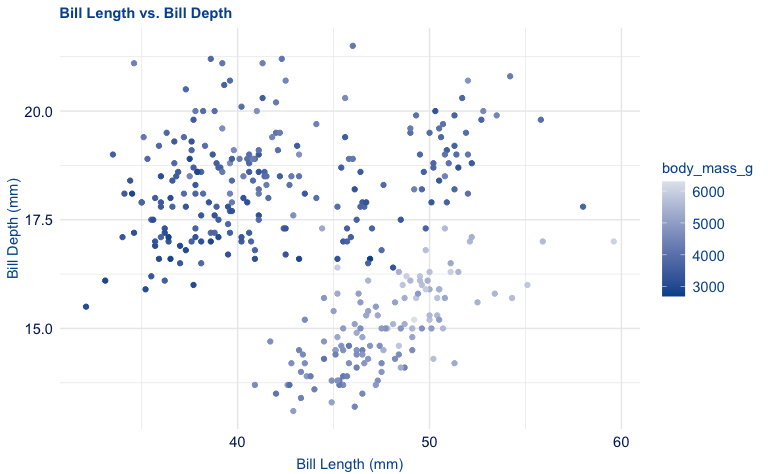``````
plot +
geom_point(aes(shape = species)) +
scale_duke_continuous() +
theme_duke()``````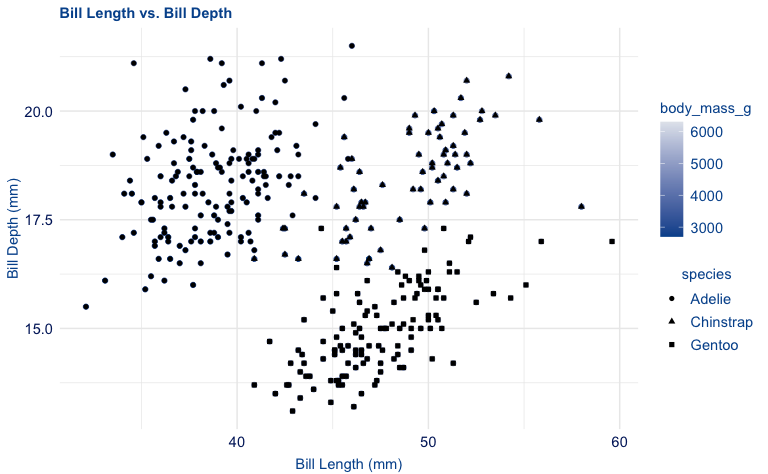``````
plot +
scale_duke_continuous() +
theme_minimal()``````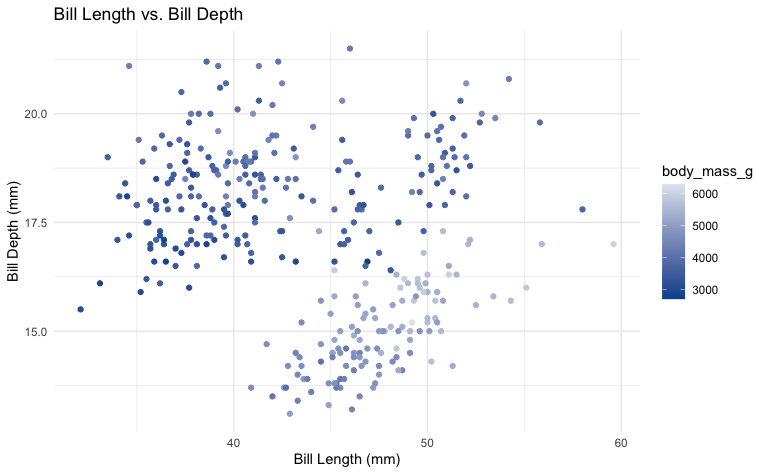## Scatter Plot - Discrete Color

``````plot1 <- ggplot(penguins, aes(x = bill_length_mm, y = bill_depth_mm, color = species)) +
geom_point(size = 2) +
labs(title = "Bill Length vs. Bill Depth", x = "Bill Length (mm)", y = "Bill Depth (mm)")

ggplot(penguins, aes(x = bill_length_mm, y = bill_depth_mm)) +
geom_point(aes(color = species)) +
labs(
title = "Bill Length vs. Bill Depth",
subtitle = "This is the subtitle",
caption = "All text is in Duke Royal Blue",
x = "Bill Length (mm)",
y = "Bill Depth (mm)"
) +
facet_wrap(~species) +
theme_duke() +
scale_duke_color_discrete()``````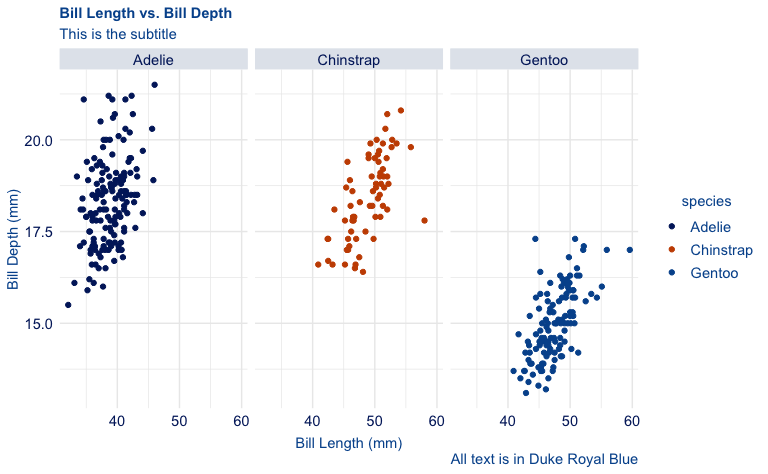``````
plot1 +
theme_duke() +
scale_duke_color_discrete()``````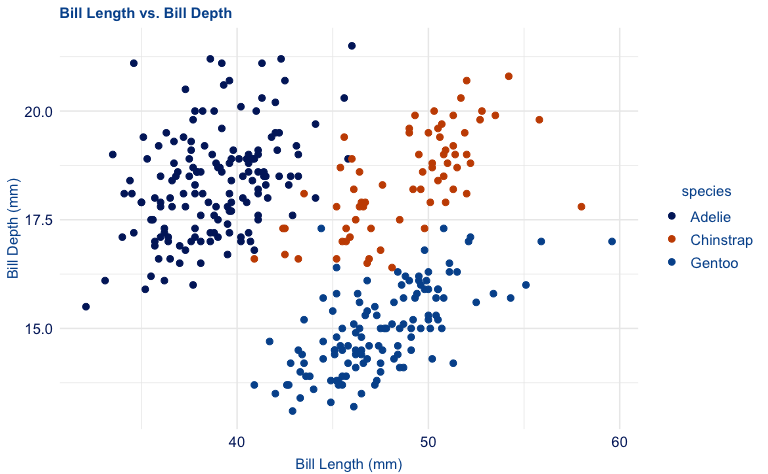``````
plot1 +
scale_duke_color_discrete() +
theme_minimal()``````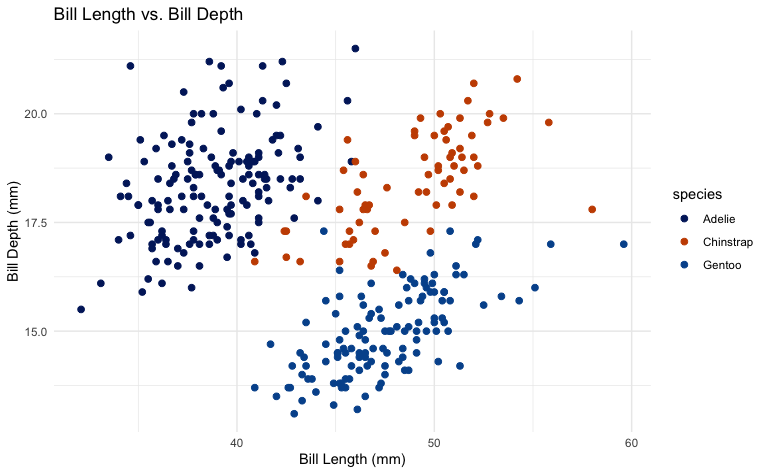## Bar Plot

``````plot2 <- ggplot(penguins, aes(x = species, fill = species)) +
geom_bar() +
labs(title = "Distribution of Penguin Species", x = "Species", y = "Count")

m_penguins <- penguins %>%
dplyr::filter(sex == "male")

plot2.1 <- ggplot(m_penguins, aes(x = sex, fill = sex)) +
geom_bar()

plot2.1 +
scale_duke_fill_discrete() +
theme_duke()``````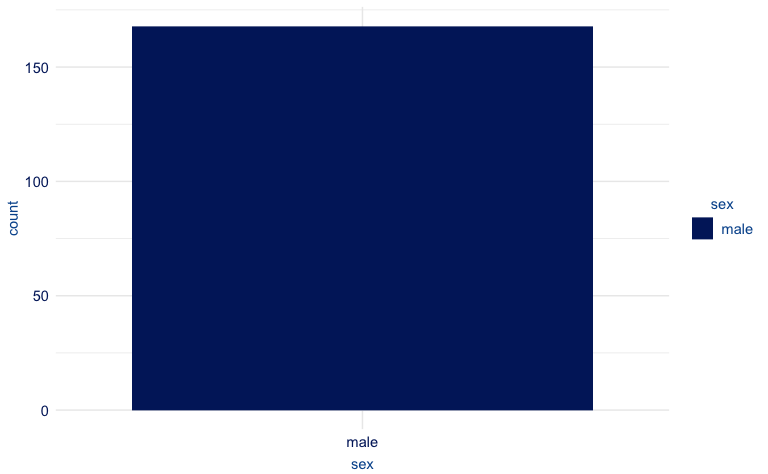``````

# 8-category plot
geom_bar()

plot2.2 +
scale_duke_fill_discrete() +
theme_duke()``````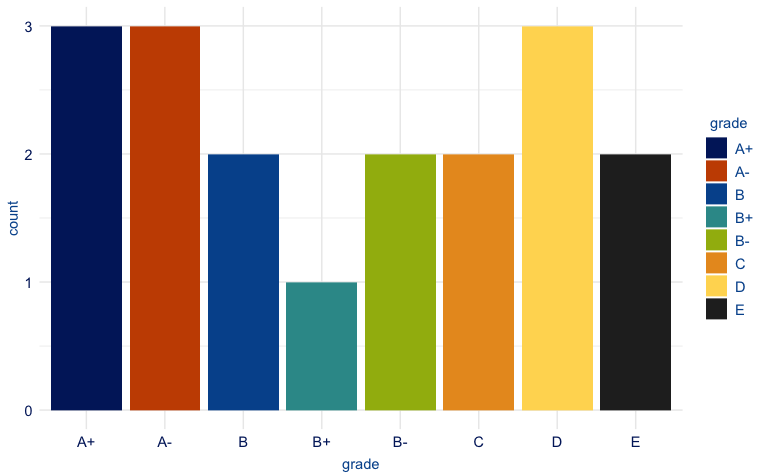``````
# 7-category plot
plot2.3 <- students %>%
slice(-13) %>%
geom_bar()

plot2.3 +
scale_duke_fill_discrete() +
theme_duke()``````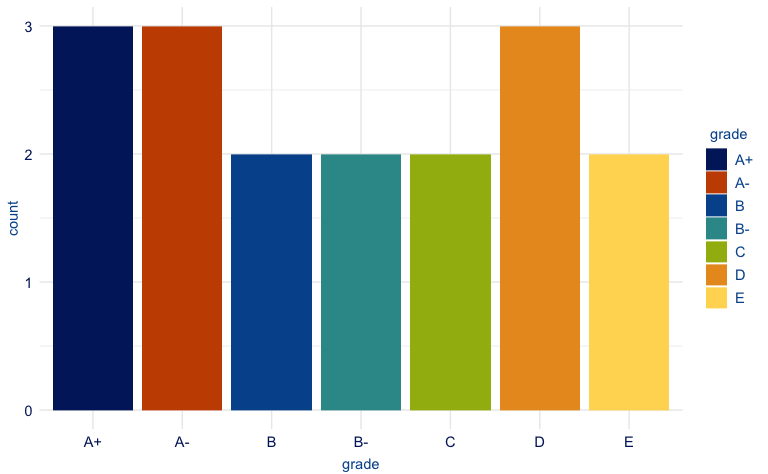``````
# 6-category plot
plot2.4 <- students %>%
slice(-c(9, 10, 13)) %>%
geom_bar()

plot2.4 +
scale_duke_fill_discrete() +
theme_duke()``````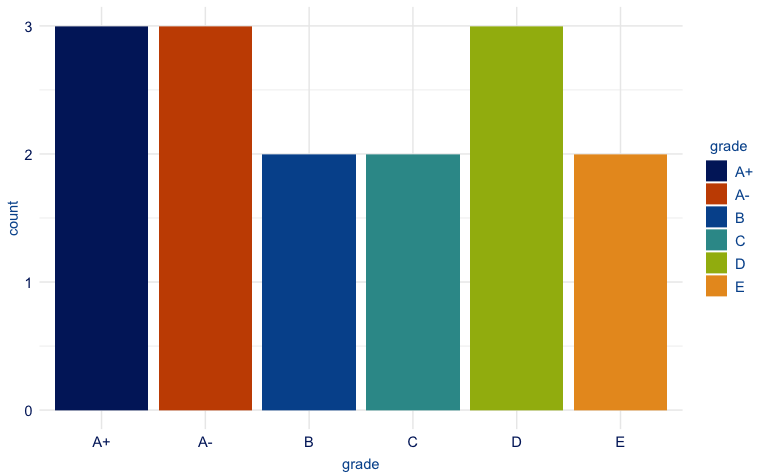``````
# 5-category plot
plot2.4 <- students %>%
slice(-c(9, 10, 13, 7, 18)) %>%
geom_bar()

plot2.4 +
scale_duke_fill_discrete() +
theme_duke()``````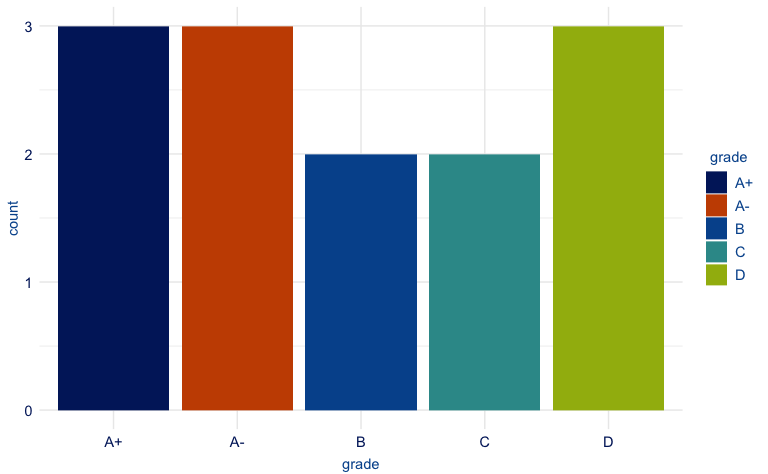``````
# 4-category plot
plot2.5 <- students %>%
slice(-c(9, 10, 13, 7, 18, 4, 8)) %>%
geom_bar()

plot2.5 +
scale_duke_fill_discrete() +
theme_duke()``````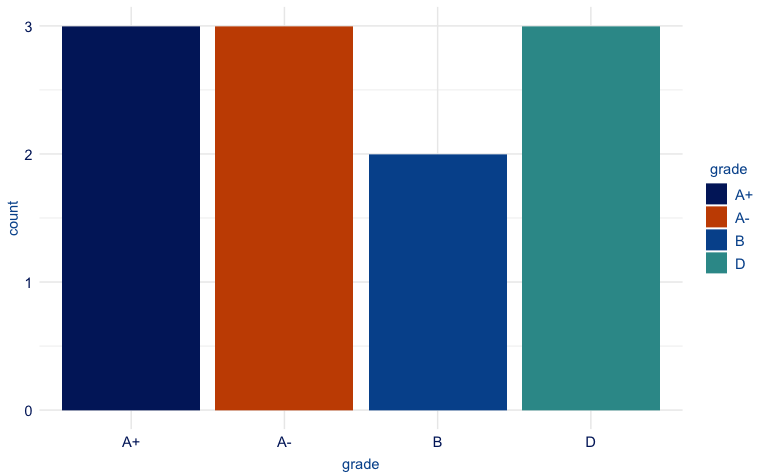## Histogram

``````plot3 <- ggplot2::ggplot(penguins, aes(body_mass_g)) +
geom_histogram(ggplot2::aes(fill = species), alpha = 0.8) +
labs(title = "Distribution of Penguin Body Mass", caption = "(Colors used) \n Duke Royal Blue, Duke Navy Blue, Copper", x = "Body Mass (g)", y = "Count")

plot3 +
scale_duke_fill_discrete() +
theme_duke()
#> `stat_bin()` using `bins = 30`. Pick better value with `binwidth`.``````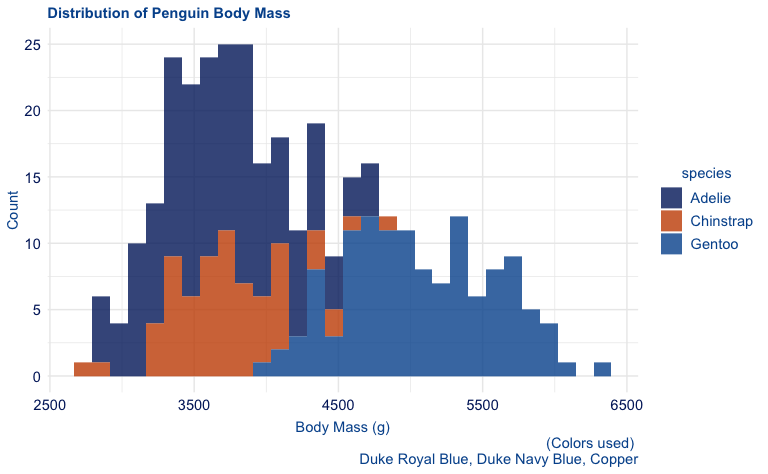``````
plot3 +
scale_duke_fill_discrete() +
theme_minimal()
#> `stat_bin()` using `bins = 30`. Pick better value with `binwidth`.``````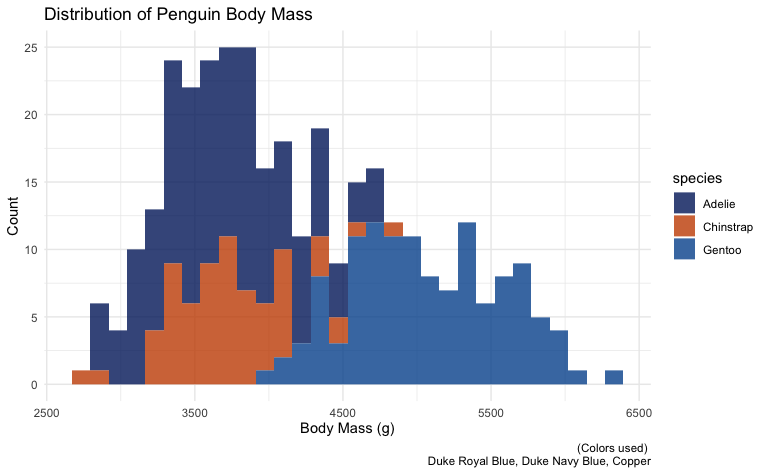## Box Plot

``````plot4 <- ggplot2::ggplot(penguins, ggplot2::aes(sex, body_mass_g)) +
ggplot2::geom_boxplot() +
ggplot2::labs(title = "Comparison of Body Mass By Sex", x = "Sex", y = "Body Mass (g)")

plot4 +
theme_duke()``````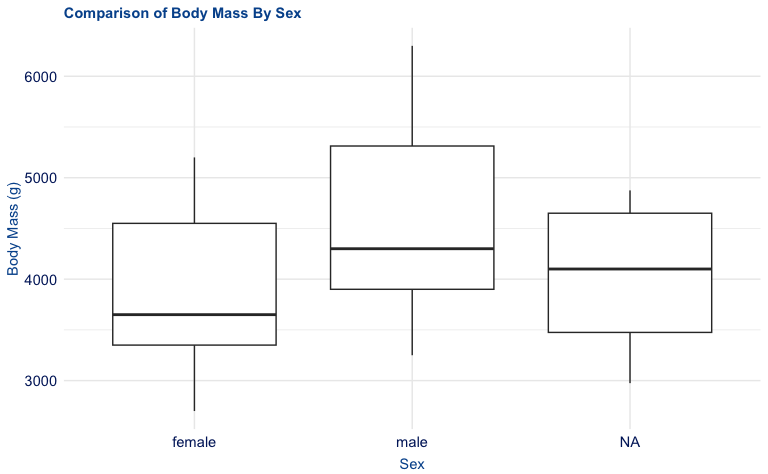``````
plot4 +
theme_minimal()``````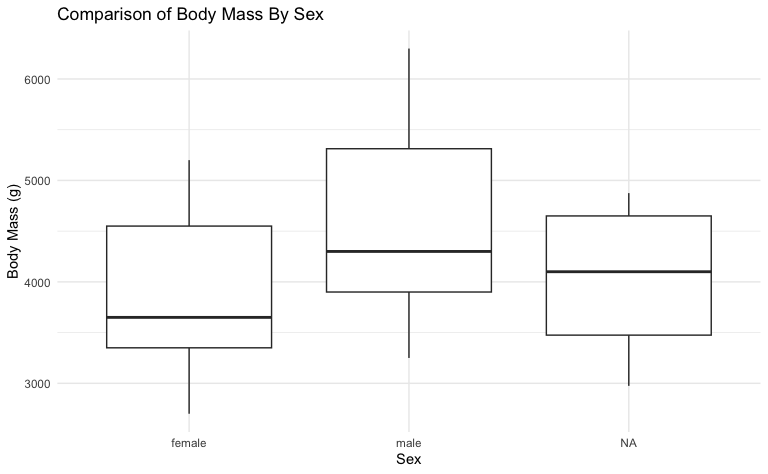## Density Plot

``````plot5 <- ggplot2::ggplot(penguins, ggplot2::aes(bill_depth_mm)) +
ggplot2::geom_density(ggplot2::aes(fill = species)) +
ggplot2::labs(title = "Density of Penguin Bill Depth", x = "Bill Depth (mm)", y = "Densiy")

plot5 +
scale_duke_fill_discrete() +
theme_duke()``````